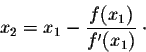# Approximating Pi using Newton-Raphson

• B
• whit3r0se-

#### whit3r0se-

Ever since the discovery of Pi, Mathematicians have been obsessed with finding methods of approximating Pi. I think I've a unique way of doing so via the Newton-Raphson.
Newton-Raphson Formula:Let ## ƒ(x)=Sin(x) ⇒ ƒ'(x)=Cos(x) ⇒ X_n= X_{n-1} - tan(X_{n-1})##
For example: Let ##X_0=X ⇒ X_3= (X-tan(X)-tan(X-tan(X))) - tan(X-tan(X)-tan(x-tan(X)))##
This cannot be calculated without a calculator therefore we can introduce a taylor expansion:
##Tan(x)≈x+x^3/3+(2 x^5)/15+(17 x^7)/315+...##
NOTE: For this to work the value of ##X_0 ## must be close to Pi, the rate of convergence is proportional to how close your value of ##X_0## is to Pi.
I'd appreciate any feedback on this method and ways to simplify/generalise my equation.

Last edited by a moderator:
Ever since the discovery of Pi, Mathematicians have been obsessed with finding methods of approximating Pi. I think I've a unique way of doing so via the Newton-Raphson.
Newton-Raphson Formula:Let ## ƒ(x)=Sin(x) ⇒ ƒ'(x)=Cos(x) ⇒ X_n= X_{n-1} - tan(X_{n-1})##
For example: Let ##X_0=X ⇒ X_3= (X-tan(X)-tan(X-tan(X))) - tan(X-tan(X)-tan(x-tan(X)))##
This cannot be calculated without a calculator therefore we can introduce a taylor expansion:
##Tan(x)≈x+x^3/3+(2 x^5)/15+(17 x^7)/315+...##
Why is this necessary? In the Newton-Raphson formula the term that is subtracted requires division. This can't reasonably be calculated without the use of a calculator unless you stipulate that all computations be performed using pencil and paper.

In your example, you say "Let ##X_0 = X##" and then immediately write something about X3. There are two problems with this:
• You have specified either X0 or X, so what good is it to set either one to the other?
• After setting X0 to X (whatever that is supposed to mean), you write an expression for X3. What happened to X1 and X2?
whit3r0se- said:
NOTE: For this to work the value of ##X_0 ## must be close to Pi, the rate of convergence is proportional to how close your value of ##X_0## is to Pi.
I'd appreciate any feedback on this method and ways to simplify/generalise my equation.

Why is this necessary? In the Newton-Raphson formula the term that is subtracted requires division. This can't reasonably be calculated without the use of a calculator unless you stipulate that all computations be performed using pencil and paper.

In your example, you say "Let ##X_0 = X##" and then immediately write something about X3. There are two problems with this:
• You have specified either X0 or X, so what good is it to set either one to the other?
• After setting X0 to X (whatever that is supposed to mean), you write an expression for X3. What happened to X1 and X2?
Well, i put ##X_3## there so people could test it out, it converges very quickly. I didn't bother writing out ## X_2 and X_1## because its fairly simple to calculate. ##X_0## refers to the original input of the formula. And as to why this is necessary, its not. There are many approximations available to use in Mathematics, but thinking of a new and creative method for another one is somehow discouraged on this forum? Pretty poor attitude.
FOR completeness:
##X_1=X-tan(X)##
##X_2=X-tan(X)-tan(X-tan(X))##

Well, i put ##X_3## there so people could test it out, it converges very quickly.
My point is that instead of saying "Let X0 = X", you should start X0 off with a specific value, such as 1, 2, -7, whatever.
whit3r0se- said:
I didn't bother writing out ## X_2 and X_1## because its fairly simple to calculate.
Not if you don't know what X0 is.
whit3r0se- said:
##X_0## refers to the original input of the formula. And as to why this is necessary, its not. There are many approximations available to use in Mathematics, but thinking of a new and creative method for another one is somehow discouraged on this forum? Pretty poor attitude.
The only part of what you did that seems new or creative is rewriting the quotient in Newton-Raphson as ##\tan(X_{n - 1})##, and then replacing it by a few terms in the Maclaurin series for the tangent function. Possibly this is the creative part, but it seems much simpler to just use some device to do the calculation, which will probably give a more precise result.

If you post something "new and creative" here, be prepared for a critique of what you post. Here is a summary of the things I see:
• Newton-Raphson is used to find a root of an equation. Nowhere in your post did you explain that you are using this technique to find a particular root of ##\sin(x) = 0##; namely, the one at ##(\pi, 0)##.
• Newton-Raphson, like most root-finding algorithms has to be "seeded" with a starting value for x0. You didn't mention starting with a value near ##\pi## until the end of your post.
• I don't see how replacing tan(x) by its Maclaurin series is any help. In your formula for X3 you have to calculate tangent of the tangent of the tangent of X0 in one place. IOW, the function composition is nested three levels, so replacing tan(x) by ##x + \frac{x^3} 3 + \dots##, and then taking the tangent of that would be problematic, not to mention taking the tangent of that.
• Finally, what happens if you don't start off with a value close to ##\pi##? Newton-Raphson is notorious for giving bad results if the graph of the function whose root you're trying to find doesn't cross the x-axis steeply or if you start off with an initial value that isn't close enough to the root you're looking for. For example, if you start off with x0 = 1.6, Newton-Raphson converges to a root of the equation sin(x) = 0 that's pretty far away from ##(\pi, 0)##.

Agree with some of your points but I recommend you actually test this out. It converges super quick.